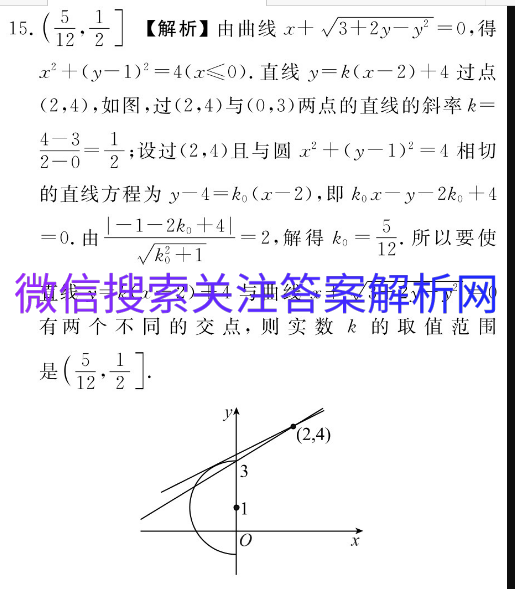# 炎德炎德长沙一中物理二

2021-01-09 17:545115.(12【解析】由曲线x+√3+2y2=0,得x2+(y-1)2=4(x≤0).直线y=k(x-2)+4过点(2,4),如图,过(2,4)与(0,3)两点的直线的斜率k=4-312-0=2;设过(2,4)且与圆x2+(y-1)2=4相切的直线方程为y-4=ko(x-2),即kx-y-2k+4=0.由-1-2k+41=2,解得k=.所以要使√k+1直线y=k(x-2)+4与曲线x+√3+2y-y2=0有两个不同的交点,则实数k的取值范围是5112’2(2,4)